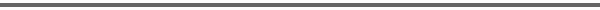Journal Home Page Complete Contentsof this Volume Previous Article Next Article Journal of Lie Theory 14 (2004), No. 2, 395--425 Copyright Heldermann Verlag 2004Variationality of Four-Dimensional Lie Group Connections Ryad Ghanam Dept. of Mathematics, University of Pittsburgh, Greensburg PA 15601, U.S.A., ghanam@pitt.edu G. Thompson Dept. of Mathematics, University of Toledo, Toledo OH 43606, U.S.A., gthomps@uoft02.utoledo.edu E.J. Miller Dept. of Mathematics, University of Toledo, Toledo OH 43606, U.S.A.Following on from previous work by one of the authors on dimensions two and three, this paper gives a comprehensive analysis of the inverse problem of Lagrangian dynamics for the geodesic equations of the canonical linear connection on Lie groups of dimension four. Starting from the Lie algebra, in every case a faithful four-dimensional representation of the algebra is given as well as one in terms of vector fields and a representation of the linear group of which the given algebra is its Lie algebra. In each case the geodesic equations are calculated as a starting point for the inverse problem. Some results about first integrals of the geodesics are obtained. It is found that in three classes of algebra, there are algebraic obstructions to the existence of a Lagrangian, which can be determined directly from the Lie algebra without the need for any representation. In all other cases there are Lagrangians and indeed whole families of them. In many cases a formula for the most general Hessian of a Lagrangian is obtained. Keywords: Canonical symmetric connection, Lie group, Lie algebra, Euler-Lagrange equations, Lagrangian, first integral of geodesics. MSC: 70H30, 70H06, 70H03, 53B40, 53C60, 57S25. [FullText-pdf (282 KB)] for subscribers only.